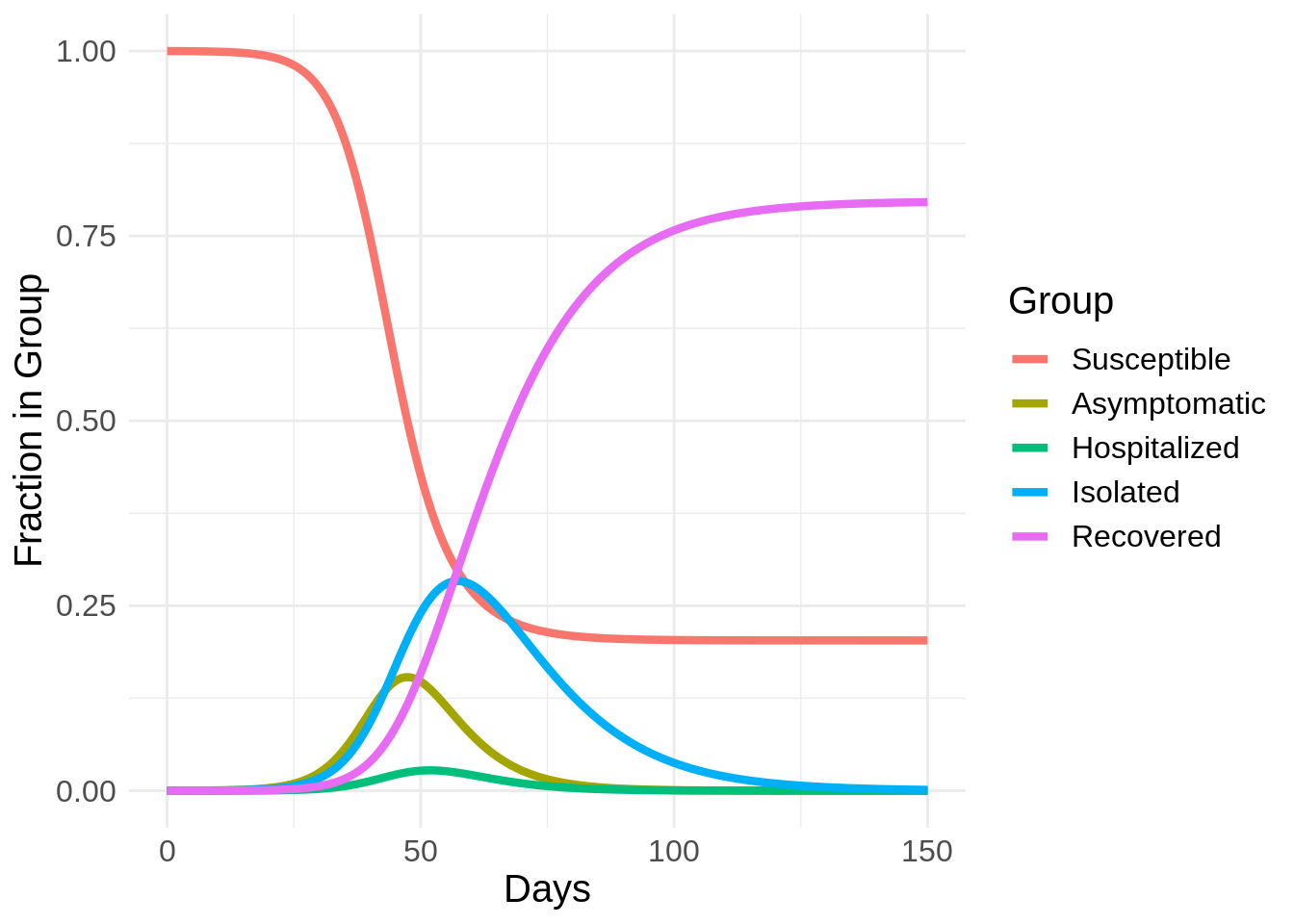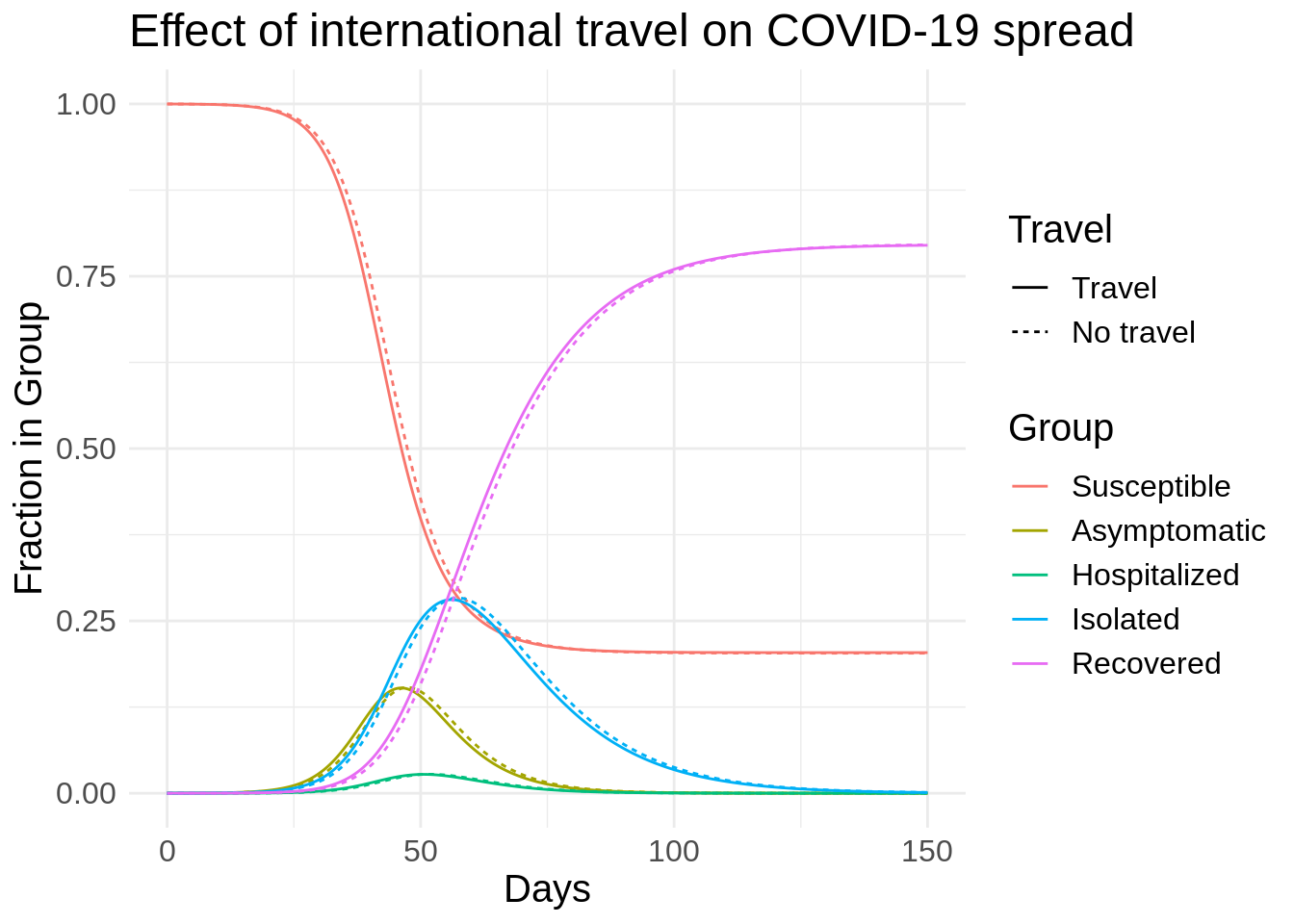# Limiting Travel Isn't Enough

Edit: a previous version of this post had the US population set as 3e11, which is 300 billion, instead of 3e8, which is 300 million. Fixing this shifts the curves to the left but does not change the interpretation of the results. Thank you Peter Farrell for the heads up.

In my previous post, I covered the SIR model and why “social distancing” is so effective at slowing infection spread and reducing healthcare system burden. In this post I’m going to cover another intervention, banning travel.

To do this, I’m going to extend the SIR model. One of the reasons that the coronavirus spreads so easily is that people can be contagious for several days before symptoms begin. So, instead of assuming three states (Susceptible, Infectious, and Recovered), I’m going to assume the infection can follow five states: Susceptible (S), Asymptomatic (A), Hospitalized (H), Isolated (I), and Recovered (R). The Susceptible become infected but Asymptomatic. The Asymptomatic eventually become ill; some become ill enough to be Hospitalized and the rest become Isolated. The Hospitalized eventually heal enough to be Isolated. Eventually the Isolated become Recovered. I assume that only the Asymptomatic can spread the virus because both the Hospitalized and Isolated are following effective quarantine measures.Flow diagram of infection

I will get to international travel later. For now, this model will allow us to break down the Infected group to more accurately model spread dynamics. This model looks like

\begin{align*} \frac{dS}{dt} &= -\beta A S \\ \frac{dA}{dt} &= \beta A S - \kappa A \\ \frac{dH}{dt} &= \kappa \eta A - \tau H \\ \frac{dI}{dt} &= \tau H + \kappa (1 - \eta) A - \phi I \\ \frac{dR}{dt} &= \phi I \end{align*}

We can interpret $$1 / \kappa$$ as the average number of days that a person is Asymptomatic, $$1 / \tau$$ as the average number of days Hospitalized, $$\eta$$ as the percent of people who require Hospitalization, and $$1 / \phi$$ as the average length of time in Isolation.

Here are the parameter values I’m using:

Parameter Value
Reproductive rate (R0) 2
Average number of days asymptomatic 5
Average number of days hospitalized 5
Percent requiring hospitalization 20%
Average number of days isolated 14
Number asymptomatic 20,000
Total population 300 million

I’ll gladly take suggestions for more accurate parameter values and I’m working on an interactive version of these plots. Nevertheless, writing this model in R and solving, we get:

library(deSolve)
library(data.table)
library(ggplot2)

sahir_ode <- function(times, init, parms) {
with(as.list(c(parms, init)), {
dS <- -beta * A * S
dA <- beta * A * S - kappa * A
dH <- kappa * eta * A - tau * H
dI <- tau * H + kappa * (1 - eta) * A - phi * I
dR <- phi * I
list(c(dS, dA, dH, dI, dR))
})
}

asymptomatic_time <- 5
hospitalized_time <- 5
hospitalized_percent <- 0.2
isolated_time <- 14

R0 <- 2
beta <- R0 / asymptomatic_time
kappa <- 1 / asymptomatic_time
eta <- hospitalized_percent
tau <- 1 / hospitalized_time
phi <- 1 / isolated_time

us_asymptomatic <- 20000
us_population <- 3e8
fraction_asymptomatic_us <- us_asymptomatic / us_population

parms <- c(beta = beta, kappa = kappa, eta = eta, tau = tau, phi = phi)
init <- c(S = 1 - fraction_asymptomatic_us, A = fraction_asymptomatic_us, H = 0, I = 0, R = 0)
times <- seq(0, 150, length.out = 2000)

sahir_out <- lsoda(init, times, sahir_ode, parms)

sahir_dt <- as.data.table(sahir_out)

group_names <- c('Susceptible', 'Asymptomatic', 'Hospitalized', 'Isolated', 'Recovered')
names(sahir_dt) <- c('time', group_names)

sahir_dt <- melt(
sahir_dt,
id.vars = c('time'),
measure.vars = group_names
)

ggplot(sahir_dt, aes(x = time, y = value, color = variable)) +
geom_line(lwd = 1.5) +
theme_minimal() +
theme(text = element_text(size = 15)) +
labs(x = 'Days', y = 'Fraction in Group', color = 'Group')I should note that even though the peak percent hospitalized here looks small, it is 2.8%. There are less than a million hospital beds in the US, which is about 0.3% of the population. So even 2.8% requiring hospitalization would result in hospitals becoming vastly overrun.

### International Travel

Now I’m going to model international travel. Let’s assume that there are two populations of roughly equal size. One has a head start on the other in terms of number of infected. Let’s assume it has five times as many asymptomatic people as the US. I’m also going to assume the same parameters as before except the other population will have 100,000 infected instead of 20,000. Each population follows the same dynamics. Travel is allowed, but only for the Susceptible, Asymptomatic, and Recovered. I’ll set a parameter $$\rho$$, which is equal to the percentage of people traveling each day. I will try out $$\rho = 0.002$$, which is roughly the proportion of the US taking international travel daily. I will compare this to $$\rho = 0$$.Flow diagram of infection spread between the two populations

Note that the results of this model will depend on the fact that the infection is already present in both populations. If one population doesn’t have the infection at all, then of course completely stopping all travel to that country will stop it from getting the disease. This is the approach that Micronesia has taken.

The more complicated model is then

\begin{align*} \frac{dS_1}{dt} &= -\beta A_1 S_1 + \rho (S_2 - S_1) \\ \frac{dA_1}{dt} &= \beta A_1 S_1 - \kappa A_1 + \rho (A_2 - A_1) \\ \frac{dH_1}{dt} &= \kappa \eta A_1 - \tau H_1 \\ \frac{dI_1}{dt} &= \tau H_1 + \kappa (1 - \eta) A_1 - \phi I_1 \\ \frac{dR_1}{dt} &= \phi I_1 + \rho (R_2 - R_1)\\ \end{align*}

\begin{align*} \frac{dS_2}{dt} &= -\beta A_2 S_2 + \rho (S_1 - S_2)\\ \frac{dA_2}{dt} &= \beta A_2 S_2 - \kappa A_2 + \rho (A_1 - A_2)\\ \frac{dH_2}{dt} &= \kappa \eta A_2 - \tau H_2 \\ \frac{dI_2}{dt} &= \tau H_2 + \kappa (1 - \eta) A_2 - \phi I_2 \\ \frac{dR_2}{dt} &= \phi I_2 + \rho (R_1 - R_2) \end{align*}

sahir_travel_ode <- function(times, init, parms) {
with(as.list(c(parms, init)), {
dS1 <- -beta * A1 * S1 + rho * (S2 - S1)
dA1 <- beta * A1 * S1 - kappa * A1 + rho * (A2 - A1)
dH1 <- kappa * eta* A1 - tau * H1
dI1 <- tau * H1 + kappa * (1 - eta) * A1 - phi * I1
dR1 <- phi * I1 + rho * (R2 - R1)

dS2 <- -beta * A2 * S2 + rho * (S1 - S2)
dA2 <- beta * A2 * S2 - kappa * A2 + rho * (A1 - A2)
dH2 <- kappa * eta * A2 - tau * H2
dI2 <- tau * H2 + kappa * (1 - eta) * A2 - phi * I2
dR2 <- phi * I2 + rho * (R1 - R2)

list(c(dS1, dA1, dH1, dI1, dR1, dS2, dA2, dH2, dI2, dR2))
})
}

simulate_sahir_travel <- function(rho) {
fraction_asymptomatic_europe <- fraction_asymptomatic_us * 5

parms <- c(beta = beta, kappa = kappa, tau = tau, phi = phi, rho = rho)
init <- c(
S1 = 1 - fraction_asymptomatic_us, A1 = fraction_asymptomatic_us, H1 = 0, I1 = 0, R1 = 0,
S2 = 1 - fraction_asymptomatic_europe, A2 = fraction_asymptomatic_europe, H2 = 0, I2 = 0, R2 = 0
)
times <- seq(0, 150, length.out = 2000)

sahir_out <- lsoda(init, times, sahir_travel_ode, parms)

sahir_dt <- as.data.table(sahir_out)

indexed_names <- as.vector(outer(group_names, c(': US', ': Europe'), FUN = paste0))
names(sahir_dt) <- c('time', indexed_names)

sahir_dt <- melt(
sahir_dt,
id.vars = c('time'),
measure.vars = indexed_names
)

sahir_dt
}

sahir_no_travel <- simulate_sahir_travel(0)
sahir_travel <- simulate_sahir_travel(0.002)

sahir_no_travel_us <- sahir_no_travel[grepl('US$', sahir_no_travel$variable)]
sahir_travel_us <- sahir_travel[grepl('US$', sahir_travel$variable)]
sahir_dt <- rbindlist(list("No travel" = sahir_no_travel_us, "Travel" = sahir_travel_us), idcol = "travel")

sahir_dt$variable <- sub(": US$", '', sahir_dt$variable) sahir_dt$variable <- factor(sahir_dt$variable, levels = group_names) sahir_dt$travel <- factor(sahir_dt\$travel, levels = c('Travel', 'No travel'))

ggplot(sahir_dt, aes(x = time, y = value, color = variable, linetype = travel)) +
geom_line(lwd = 0.5) +
theme_minimal() +
theme(text = element_text(size = 15)) +
labs(
x = 'Days',
y = 'Fraction in Group',
color = 'Group',
linetype = 'Travel',
title = "Effect of international travel on COVID-19 spread"
)The effect of banning international travel is the shift from solid lines to dashed lines, which is minimal. The reason is that it has already spread to the United States. Even though other countries have a head start, allowing travel only shifts the trajectory of the infection spread up a few days.

There is one main drawback to this model: the effect of travel itself. When people fly, they are traveling in close quarters with others, in recycled air, and with reduced immune systems. So the act of travel itself temporarily spikes $$\beta$$ for those traveling. Nevertheless, this model shows that the most effective way to reduce the spread of the coronavirus is consistent and extended social distancing.

Huge thanks to my friend Jonathan Lieberman for several rounds of editing and working with me to fine tune the model.